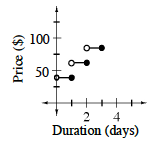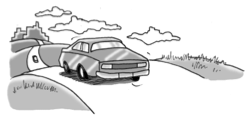### Home > CCA2 > Chapter Ch3 > Lesson 3.1.3 > Problem3-56

3-56.

In the year 2006, the average cost to rent a car was $\39$ for the first day and an additional $\23$ for each additional day. Homework Help ✎

1. Graph the relationship between cost and the duration of a car rental in 2006.

If you rent a car for half a day, three-quarters of a day, or a full day, you pay the same rate. What kind of graph is this?

For $1.5$ days, $1.75$ days, or $2$ days you will pay $\39$ for the first day and $\23$ for the second, even if you are just late bringing the car back by an hour.2. Describe how the graph would be transformed if the current average cost of a car rental has increased to $\50$ for the first day.

If you don't remember what it means to transform a graph you can look it up in the glossary.